Chin. Phys. B, 2020, Vol. 29(11): 110302    DOI: 10.1088/1674-1056/abb45b
 GENERAL Prev   Next

# Zitterbewegung of Dirac quasiparticles emerged in a Su-Schrieffer–Heeger lattice

Yue Hu(胡玥)1, Zheng-Xin Guo(郭政鑫)1, Ze-Ming Zhong(钟泽明)1,2, †, and Zhi Li(李志)1,, ‡
1 Guangdong Provincial Key Laboratory of Quantum Engineering and Quantum Materials, Guangdong Provincial Engineering Technology Research Center for Quantum Precision Measurement, SPTE, South China Normal University, Guangzhou 510006, China
2 School of Materials Science and Engineering, Guangdong Provincial Key Laboratory of Advanced Energy Storage Materials, South China University of Technology, Guangzhou 510641, China
 Abstract  We analytically and numerically investigate the dynamical properties of the tilted dispersion relativistic quasiparticles emerged in a cold atomic optical lattice system. By introducing the next nearest neighboring (NNN) hopping term into Su–Schrieffer–Heeger (SSH) model, the Dirac quasiparticles with tilted dispersion relation are realized. The results show that the tilted dispersion causes a drift in relativistic quasiparticles rather than affecting interference behavior between inner states. To be specific, the relativistic phenomena of the quasiparticles induced by the inner state interference (such as Zitterbewegung, Klein paradox, etc.) is completely unaffected by the tilted dispersion. In order to distinguish the drift induced by tilted dispersion and common initial velocity, we calculate the momentum distribution of the relativistic quasiparticles. We obtain the difference between the drift induced by initial velocity and tilted dispersion. The former affects the ZB, while the latter does not. By using this character, we propose a quench dynamics scheme to obtain a stable mono-spin state. The proposed cold atomic lattice system would provide a promising platform in exploring the intrinsic exotic physics of relativistic quasiparticles and the related systems. Keywords:  tilted dispersion      wave-packet dynamics      quantum quench Received:  09 June 2020      Revised:  23 July 2020      Accepted manuscript online:  02 September 2020 Fund: the National Key Research and Development Program of China (Grant Nos. 2016YFA0301803 and 2016YFA0302800), the National Natural Science Foundation of China (Grant Nos. 11604103, 11704132, 11822403, and 91636218), the NSAF, China (Grant Nos. U1801661 and U1830111), the PCSIRT, China (Grant No. IRT1243), the Natural Science Foundation of Guangdong Province, China (Grant Nos. 2016A030313436, 2018A030313322, and 2018A0303130066), the KPST of Guangzhou (Grant No. 201804020055), China Postdoctoral Science Foundation (Grant No. 2018M633063), and the Startup Foundation of SCNU. Corresponding Authors:  †Corresponding author. E-mail: zhongzeming_scut@163.com ‡Corresponding author. E-mail: lizhi_nju@163.com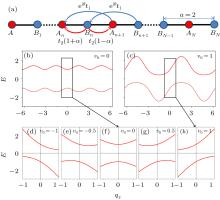Fig. 1.  (a) The proposed one-dimensional chain optical lattice scheme and atomic hopping configuration between the different A (or B) sublattice sites. t1 (t2) denotes the hopping between sites of the same (different) type atoms, a = 1 is the lattice constant. θ = π/2 is the hopping phase factor. (b) and (c) Dispersion relationship in the condition of Bloch Hamiltonian with v0 = 0 and v0 = 1, respectively. (d)–(h) Dispersion relationship with different v0 in the condition of the low-energy effective Hamiltonian [Eq. (5)].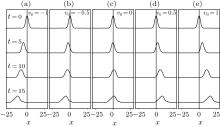Fig. 2.  (a)–(e) Numerically calculated probability distributions, |Ψ(x,t)|2, with different time. The width of the initial wave packet L = 2, effective mass m = 1, effective light speed vx = 1.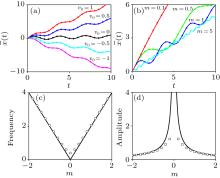Fig. 3.  Analytically (lines) and numerically (symbols) calculated ZB effect. The expectation value $\bar{x}(t)$ with different v0 (m) is shown in (a) [(b)]. The corresponding frequency (c) and amplitude (d) of ZB with different m are provided. Throughout the calculation, the width of the initial wavepacket L = 2 and effective fermi velocity vx = 1.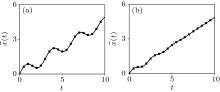Fig. 4.  The expectation value $\bar{x}(t)$ for the cases of (a) v0 = 0.5 and (b) q0 = 0.5. The lines (symbols) correspond to the exact solution (numerical results).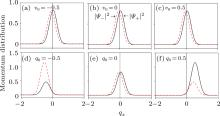Fig. 5.  Momentum distributions of the positive and negative energy parts of the wave packet: (a) v0 = −0.5, (b) v0 = 0, (c) v0 = 0.5 with q0 = 0; (d) q0 = −0.5, (e) q0 = 0, (f) q0 = 0.5 with v0 = 0.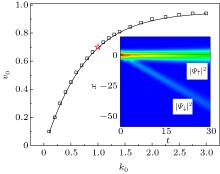Fig. 6.  The relationship of q0 and v0 for a stable single pure component wave packet. Insert: Visualized wavepacket for the condition of red star. Through the numerical calculation, the other parameters L = 2, q0 = 0.5, m = 1, and vx = 1.